## The decibel scale

The ear mechanism is able to respond to both very small and very large pressure waves by virtue of being nonlinear; that is, it responds much more efficiently to sounds of very small amplitude than to sounds of very large amplitude. Because of the enormous nonlinearity of the ear in sensing pressure waves, a nonlinear scale is convenient in describing the intensity of sound waves. Such a scale is provided by the sound intensity level, or decibel level, of a sound wave, which is defined by the equationBritannica Quiz
Sound Waves Calling
What is another name for the voice box?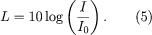Here L represents decibels, which correspond to an arbitrary sound wave of intensity I, measured in watts per square metre. The reference intensity I0, corresponding to a level of 0 decibels, is approximately the intensity of a wave of 1,000 hertz frequency at the threshold of hearing—about 10-12 watt per square metre. Because the decibel scale mirrors the function of the ear more accurately than a linear scale, it has several advantages in practical use; these are discussed in Hearing, below.

A fundamental feature of this type of logarithmic scale is that each unit of increase in the decibel scale corresponds to an increase in absolute intensity by a constant multiplicative factor. Thus, an increase in absolute intensity from 10-12 to 10-11 watt per square metre corresponds to an increase of 10 decibels, as does an increase from 10-1 to 1 watt per square metre. The correlation between the absolute intensity of a sound wave and its decibel level is shown in Table 1, along with examples of sounds at each level. When the defining level of 0 decibel (10-12 watt per square metre) is taken to be at the threshold of hearing for a sound wave with a frequency of 1,000 hertz, then 130 decibels (10 watts per square metre) corresponds to the threshold of feeling, or the threshold of pain. (Sometimes the threshold of pain is given as 120 decibels, or 1 watt per square metre.)

Sound levels for nonlinear (decibel) and linear (intensity) scales
decibels intensity* type of sound
*In watts per square metre.
130 10 artillery fire at close proximity (threshold of pain)
120 1 amplified rock music; near jet engine
110 10−1 loud orchestral music, in audience
100 10−2 electric saw
90 10−3 bus or truck interior
80 10−4 automobile interior
70 10−5 average street noise; loud telephone bell
60 10−6 normal conversation; business office
50 10−7 restaurant; private office
40 10−8 quiet room in home
30 10−9 quiet lecture hall; bedroom
20 10−10 radio, television, or recording studio
10 10−11 soundproof room
0 10−12 absolute silence (threshold of hearing)

Although the decibel scale is nonlinear, it is directly measurable, and sound-level meters are available for that purpose. Sound levels for audio systems, architectural acoustics, and other industrial applications are most often quoted in decibels.

## In gases

For longitudinal waves such as sound, wave velocity is in general given as the square root of the ratio of the elastic modulus of the medium (that is, the ability of the medium to be compressed by an external force) to its density: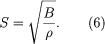Here ρ is the density and B the bulk modulus (the ratio of the applied pressure to the change in volume per unit volume of the medium). In gas mediums this equation is modified to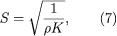where K is the compressibility of the gas. Compressibility (K) is the reciprocal of the bulk modulus (B), as in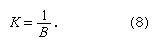Using the appropriate gas laws, wave velocity can be calculated in two ways, in relation to pressure or in relation to temperature: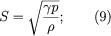or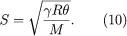Here p is the equilibrium pressure of the gas in pascals, ρ is its equilibrium density in kilograms per cubic metre at pressure p, θ is absolute temperature in kelvins, R is the gas constant per mole, M is the molecular weight of the gas, and γ is the ratio of the specific heat at a constant pressure to the specific heat at a constant volume,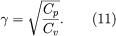Values for γ for various gases are given in many physics textbooks and reference works. The speed of sound in several different gases, including air, is given in Table 2.

Speed of sound in selected gases
gas speed
metres/second feet/second
helium, at 0 °C (32 °F) 965 3,165
nitrogen, at 0 °C 334 1,096
oxygen, at 0 °C 316 1,036
carbon dioxide, at 0 °C 259 850
air, dry, at 0 °C 331.29 1,086
steam, at 134 °C (273 °F) 494 1,620

Equation (10) states that the speed of sound depends only on absolute temperature and not on pressure, since, if the gas behaves as an ideal gas, then its pressure and density, as shown in equation (9), will be proportional. This means that the speed of sound does not change between locations at sea level and high in the mountains and that the pitch of wind instruments at the same temperature is the same anywhere. In addition, both equations (9) and (10) are independent of frequency, indicating that the speed of sound is in fact the same at all frequencies—that is, there is no dispersion of a sound wave as it propagates through air. One assumption here is that the gas behaves as an ideal gas. However, gases at very high pressures no longer behave like an ideal gas, and this results in some absorption and dispersion. In such cases equations (9) and (10) must be modified, as they are in advanced books on the subject.

## In liquids

For a liquid medium, the appropriate modulus is the bulk modulus, so that the speed of sound is equal to the square root of the ratio of the bulk modulus (B) to the equilibrium density (ρ), as shown in equation (6) above. The speed of sound in liquids under various conditions is given in Table 3. The speed of sound in liquids varies slightly with temperature—a variation that is accounted for by empirical corrections to equation (6), as is indicated in the values given for water in Table 3.

Speed of sound in selected liquids
(at one atmosphere pressure)
liquid speed
metres/second feet/second
pure water, at 0 °C (32 °F) 1,402.3 4,600
pure water, at 30 °C (86 °F) 1,509.0 4,950
pure water, at 50 °C (122 °F) 1,542.5 5,060
pure water, at 70 °C (158 °F) 1,554.7 5,100
pure water, at 100 °C (212 °F) 1,543.0 5,061
salt water, at 0 °C 1,449.4 4,754
salt water, at 30 °C 1,546.2 5,072
methyl alcohol, at 20 °C (68 °F) 1,121.2 3,678
mercury, at 20 °C 1,451.0 4,760

## In solids

For a long, thin solid the appropriate modulus is the Young’s, or stretching, modulus (the ratio of the applied stretching force per unit area of the solid to the resulting change in length per unit length; named for the English physicist and physician Thomas Young). The speed of sound, therefore, is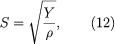where Y is the Young’s modulus and ρ is the density. Table 4 gives the speed of sound in representative solids.

Speed of sound in selected solids
solid speed
metres/second feet/second
aluminum, rolled 5,000 16,500
copper, rolled 3,750 12,375
iron, cast 4,480 14,784
Pyrex™ 5,170 17,061
Lucite™ 1,840 6,072

In the case of a three-dimensional solid, in which the wave is traveling outward in spherical waves, the above expression becomes more complicated. Both the shear modulus, represented by η, and the bulk modulus B play a role in the elasticity of the medium: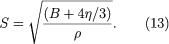Sound

## External Websites

Britannica Websites
Articles from Britannica Encyclopedias for elementary and high school students.

## Article Contributors

×
Britannica presents a time-travelling voice experience